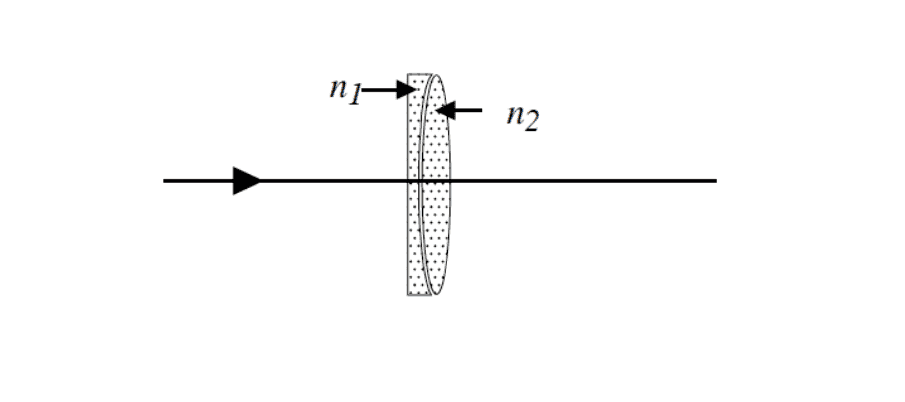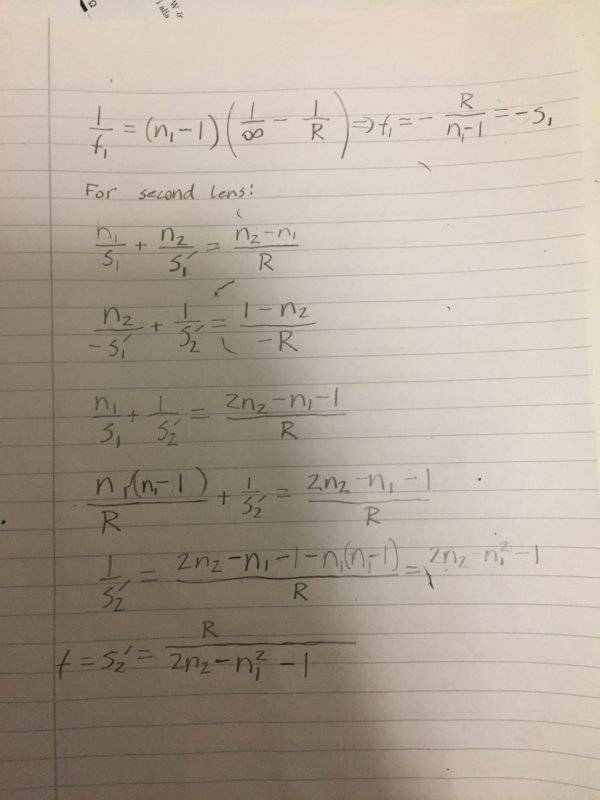# Calculate the focal distance of the duplicate

Karl Karlsson
Homework Statement:
Calculate the focal distance of the duplicate
Relevant Equations:
Lensmaker's equation
The picture below shows a so-called chromatic doublet, which is designed to minimize chromatic aberration, ie the wavelength dependence of the refractive index of the glass. The first lens has a flat first surface and a concave second surface with radius of curvature R and index of refraction n1 . The second lens is double convex with curvature radius R (refraction index n2) and sits close to the first lens. The lenses can be considered thin.Calculate the focal distance of the duplicate

My try:I seem to be getting the wrong answer. What am i doing wrong?

Correct answer is R/(2*n2 - n1 - 1)

#### Attachments

Homework Helper
Gold Member
What did you use for the focal length of the second lens? Note that the figure shows a clear air gap between the two lenses. This means that you should find the two focal lengths as if the lenses were surrounded by air.

On edit: You need to adopt the standard strategy for solving compound lens problems of this kind: (a) Put an object at some finite distance ##s## in front of the combination; (b) find the position of the image ##s'##; (c) Treat the image as the object for the second lens (pay close attention to what is real and what is virtual); (d) find the position of the final image ##s''##; (e) let ##s## go to infinity and see what ##s''## becomes; (f) relate ##s''## to the focal length of the combination.

I tried this method for this problem and got the answer you quoted as correct.

Last edited: